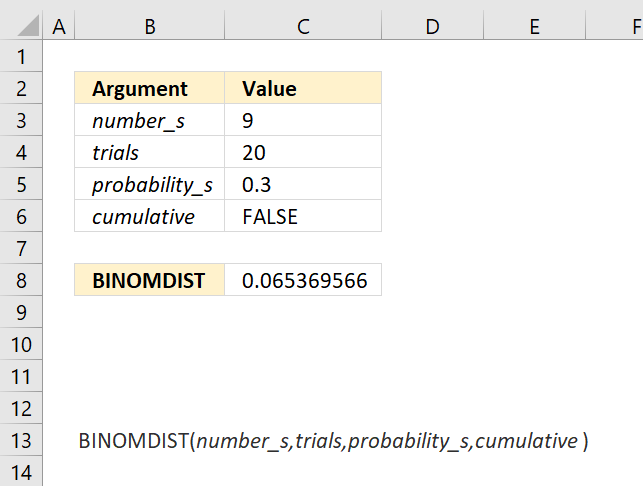Author: Oscar Cronquist Article last updated on May 11, 2022The BINOMDIST function calculates the individual term binomial distribution probability, use this function when

• the success probability is constant through all trials
• you know the number of trials
• the outcome is either a success or failure
• each trial is independent of the other trials.

The BINOMDIST function is outdated and replaced by the BINOM.DIST function.

Formula in cell C8:

=BINOMDIST(C3,C4,C5,C6)

### Excel Function Syntax

BINOMDIST(number_s,trials,probability_s,cumulative)

### Arguments

 number_s Required. The number of successful tests. trials Required. How many independent tests. probability_s Required. The probability of success in each test. cumulative Required. A boolean value. TRUE - cumulative distribution function FALSE - probability mass function